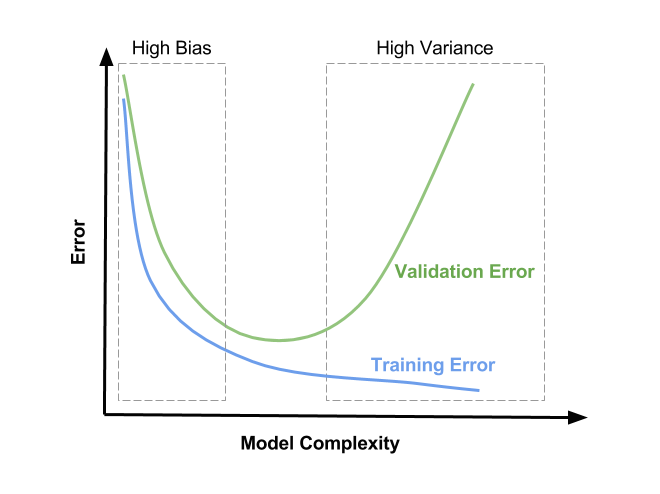## To err is human, to forgive divine, how about when it comes to machine

AI Insights

All people commit sins and make mistakes. God forgives them, and people are acting in a godlike way when they forgive. This saying was from “An Essay on Criticism” by Alexander Pope.

The machines are invented by a human, of course, they err as well but, how much does it err? In machine learning or data science, we force the machine to understand the relationship between two types of variables, which are dependent variable (Y) and independent variables (X). For example, your math score (Y) depends on the average hours spent studying math per week (X¹) and the average hours spent on Facebook per day (X²).

How to find the relationship between these three?

Let f(X¹, X²) be the function of X¹ and X² which describes the relationship by,

Y = f(X¹, X²) + ϵ

What does ϵ (epsilon) mean?

It means noise/residual of the system, the unexplained variability outside the scope of our function f(X¹, X²), which is one of the three errors in the output of the machines learning model — bias, variance, and noise.

Statistically speaking, we must assume that residuals are independent and identically normally distributed with zero mean and standard deviation equals σᵣ, otherwise all of those assumptions are invalid.

In real-world problems, it is impossible to know how such a f(X¹, X²) looks like. We have to estimate it and for the sake of mathematical modeling, we are going to estimate f(X¹, X²) by employing g(X¹, X²). Please keep in mind that every estimation comes with errors.

Now we have an assumption and ready to start training and testing it out!

After sampling, we have a sample size equal to n, {(y¹, x¹¹, x²¹), (y², x¹², x²²), …, (yⁿ, x¹ⁿ, x²ⁿ)}.

We partition the sample into two sets, a training set, and a test set. The training set is trained according to the assumption, g(x¹, x²).

After the machines learned and understood the relationship, now they can return us the predicted value of Y by utilizing g(x¹, x²).

Basically, good estimations/predictions come from tiniest differences between actual values (y) and predicted/estimated values g(x¹, x²). This difference is conventionally called “errors” (now you know how machines err!), and the popular metric to measure errors is “mean-squared error” or the expected value (𝔼)of (y -g(x¹, x²))² and it should ideally converge to zero.

𝔼[(y -g(x¹, x²))²] = 𝔼[f(x¹, x²) -g(x¹, x²)]² -(𝔼[g(x¹, x²)²] -𝔼[g(x¹, x²)]²)-σᵣ²

Or

### Err(x) = Bias² + Variance + noise

You can have many assumptions {g¹(x¹, x²), g²(x¹, x²), …, gᵏ(x¹, x²)}. However, all assumptions have to go through the evaluation process. To evaluate assumptions (or technically called models), cross-validation method is used. Precision, Recall, Accuracy and F1 score from validating test set are the most famous metrics to evaluate the performance of the models.

Testing out the assumptions helps us answer which model is the most suitable and good enough for the tasks. Furthermore, those metrics indirectly tell us the about bias and variance of the model. For instance, if the metrics are low only on test set then the model tends to overfit. See the plot for more details:In conclusion, the term errors in machines learning are decomposed into three terms: bias, variance and, noise.

Bias is the difference between assumptions, g(x¹, x²) and the actual system, f(x¹, x²). On the other hand, Variance means how much the assumptions work distinctively on samples (e.g., training set) and out of sample data sets (e.g., test set).

Bias and variance have an inverse relationship. To lower bias is to force variance to go higher and vice versa. For more details please see this bias-variance tradeoff.

Finally, the last composition of error — “noises”. Machines learning practitioners should ignore and avoid to focus on this error. Noises should not be allowed to bend in with the other factors in the assumptions as noise unnecessarily disturbs the relationships.